Select Page

# Semiconductor Diode

Semiconductor Diode

Contents

Recall P-N Junction Diode 1

Describe Biasing of P-N Junction Diode 1

Describe V-I characteristics of P-N Junction Diode 3

Recall the Diode Switching time and Rating 4

Recall the Current Flow Mechanism in P-N Junction Diode 5

Derive the expression of Current in P-N Junction Diode 5

Describe the effect of Temperature on Barrier Potential 7

Describe the effect of Temperature on Leakage Current 7

Describe the Diode C-V Characteristics 7

Recall the Static and Dynamic Resistances of Diode 7

Recall the Piecewise Linear Model of a Diode 7

Recall the Diode Approximation Model 7

Describe the Small-signal Model of a Diode 7

Describe the Large-signal Model of a Diode 7

Describe the Half-Wave Rectifier 7

Derive the parameters of Half-Wave Rectifier i. Average and RMS values of Voltage and Current ii. Form Factor and Ripple Factor iii. Efficiency and Peak Inverse Voltage 7

Describe the Full-Wave Centre-Tap Rectifier 7

Describe the Full-Wave Bridge Rectifier 7

Derive the parameters of Full-Wave Centre-Tap and Bridge Rectifiers 7

Recall Filters 7

Describe the working of Capacitor Filter 7

Calculate the Ripple Factor of Capacitor Filter 7

Describe the working of Inductor Filter 7

Calculate the Ripple Factor of Inductor Filter 7

Describe the working of LC Filter 7

Describe the working of CLC or π-Filter 7

Describe the working of Series Clipper Circuit and draw the output waveform of the Circuit 7

Describe the working of Shunt Clipper Circuit and draw the output waveform of the Circuit 7

Describe the working of Shunt-Biased Clipper Circuit and draw the output waveform of the Circuit 7

Describe the working of Series-Biased Clipper Circuit and draw the output waveform of the Circuit 7

Recall the Combinational Clipper Circuit 7

Draw the Output Waveform of the Clipper Circuit 7

Recall the Clamper Circuits and their Types 7

Describe the working of Positive Clamper Circuit and draw the output waveform of the Circuit 7

Describe the working of Negative Clamper Circuit and draw the output waveform of the Circuit 7

Recall the biased Clamper Circuits 7

Draw the output waveform of Biased Clamper Circuits 7

Recall the Voltage Doubler Circuits 7

Describe the working of Voltage Doubler Circuits 7

Describe the Voltage Tripler Circuit 7

Describe the working of Voltage Quadrupler Circuit 7

# Recall P-N Junction Diode

A p-n junction diode is a two-terminal semiconductor device that allows electric current to flow in only one direction. It consists of a p-type semiconductor material, which has an excess of holes (positive charge carriers), and an n-type semiconductor material, which has an excess of electrons (negative charge carriers). The two materials are brought into contact to form a p-n junction.

The p-n junction acts as a rectifier, allowing current to flow in only one direction. When a voltage is applied to the p-n junction with the positive voltage on the p-type side and the negative voltage on the n-type side, a forward bias is created, and current can flow through the device. If the voltage is reversed, a reverse bias is created, and the p-n junction acts as a barrier to the flow of current.

P-n junction diodes are widely used in electronics, as they can perform a variety of functions, such as rectification, voltage regulation, and signal mixing. They are also the building blocks for more complex semiconductor devices, such as bipolar junction transistors (BJTs) and metal-oxide-semiconductor field-effect transistors (MOSFETs).

# Describe Biasing of P-N Junction Diode

Biasing a p-n junction diode refers to the process of applying an external voltage to the diode to establish a desired operating condition. The biasing of a p-n junction diode can be categorized into three types: forward bias, reverse bias, and zero bias.

Here’s a description of each biasing condition:

1. Forward Bias:
• In forward bias, the positive terminal of the voltage source is connected to the p-type side of the diode, and the negative terminal is connected to the n-type side.
• This arrangement creates a potential difference that allows the majority carriers (holes in the p-region and electrons in the n-region) to move towards the junction.
• The forward bias reduces the width of the depletion region, allowing current to flow through the diode.
• The diode exhibits low resistance in the forward bias region, and it is typically used for rectification and conducting current.
2. Reverse Bias:
• In reverse bias, the positive terminal of the voltage source is connected to the n-type side of the diode, and the negative terminal is connected to the p-type side.
• This configuration creates a potential barrier that opposes the flow of majority carriers and widens the depletion region.
• The reverse bias increases the width of the depletion region, effectively preventing significant current flow through the diode.
• However, a small reverse saturation current (due to minority carriers) can flow.
• The diode exhibits high resistance in the reverse bias region, and it is commonly used for protection, voltage multiplication, and signal modulation.
3. Zero Bias:
• Zero bias refers to the condition where no external voltage is applied to the diode.
• In this state, the diode operates based on the characteristics of the p-n junction alone.
• The depletion region width is determined by the properties of the p-n junction and the doping levels of the semiconductor material.
• The diode behaves as a natural diode, with a specific depletion region width and associated potential barrier.

It’s important to note that the biasing condition affects the behavior and characteristics of the p-n junction diode. The choice of biasing is crucial in various electronic circuits to ensure proper operation and achieve the desired functionality.

By applying appropriate biasing to a p-n junction diode, it can be utilized in various applications such as rectification, voltage regulation, signal detection, modulation, and switching. Understanding the biasing conditions and their effects is fundamental in designing and analyzing electronic circuits involving diodes.

# Describe V-I characteristics of P-N Junction Diode

The V-I (Voltage-Current) characteristics of a p-n junction diode describe the relationship between the voltage applied across the diode and the resulting current flowing through it. The V-I characteristics of a p-n junction diode can be divided into three regions: forward bias, reverse bias, and breakdown.

Here’s a description of each region:

1. Forward Bias Region:
• In the forward bias region, the positive terminal of the voltage source is connected to the p-type region of the diode, and the negative terminal is connected to the n-type region.
• As the forward bias voltage increases, it reduces the potential barrier of the p-n junction, allowing majority carriers (holes in the p-region and electrons in the n-region) to move across the junction.
• Initially, there is a small forward voltage drop across the diode called the forward voltage (Vf).
• Once the forward voltage reaches a certain threshold value (typically around 0.6-0.7 volts for silicon diodes), the diode starts conducting current rapidly.
• In the forward bias region, the diode exhibits a low resistance and allows a significant current flow.
• The current flowing through the diode in the forward bias region is an exponential function of the forward voltage.
2. Reverse Bias Region:
• In the reverse bias region, the positive terminal of the voltage source is connected to the n-type region of the diode, and the negative terminal is connected to the p-type region.
• Reverse biasing increases the potential barrier of the p-n junction, widening the depletion region and preventing the flow of majority carriers.
• Only a small reverse saturation current, resulting from minority carriers, flows in this region.
• The reverse current (IR) remains extremely low until the reverse bias voltage exceeds the breakdown voltage.
• The breakdown voltage is the voltage at which the reverse current sharply increases, and the diode enters the breakdown region.
3. Breakdown Region:
• In the breakdown region, the reverse bias voltage exceeds the breakdown voltage of the diode.
• The breakdown can occur in two forms: Zener breakdown or avalanche breakdown, depending on the diode’s construction and characteristics.
• In the breakdown region, the diode exhibits a very high reverse current and may be damaged if the current is not limited.

The V-I characteristics of a p-n junction diode can be graphically represented as follows:

• In the forward bias region, the current increases rapidly with a small change in voltage.
• In the reverse bias region, the current remains very low until the breakdown voltage is reached, at which point the current increases sharply.

Understanding the V-I characteristics of a p-n junction diode is crucial in designing and analyzing electronic circuits involving diodes. These characteristics help determine the diode’s behavior under different biasing conditions and aid in selecting appropriate diodes for specific applications.

# Recall the Diode Switching time and Rating

Diode switching time refers to the amount of time it takes for a diode to transition from its off-state to its on-state or vice versa. In other words, it is the time required for the diode to switch from blocking current flow to allowing current flow, or from allowing current flow to blocking current flow.

Diode switching time is dependent on a number of factors, including the diode’s material, its size and geometry, the voltage and current applied, and the ambient temperature. In general, switching times for diodes range from a few nanoseconds to a few microseconds.

Diode rating refers to the maximum value of voltage, current, and power that a diode can handle without damage. The voltage rating of a diode is the maximum reverse voltage that it can withstand without breaking down. The current rating of a diode is the maximum continuous forward current that it can handle without overheating and damaging the diode. The power rating of a diode is the product of the voltage rating and the current rating, and represents the maximum power that the diode can handle.(font size)

It’s important to select a diode with appropriate ratings for the specific application, as exceeding the ratings can lead to permanent damage or failure of the diode.

# Recall the Current Flow Mechanism in P-N Junction Diode

The current flow mechanism in a p-n junction diode is based on the diffusion of majority carriers (electrons in the n-type material and holes in the p-type material) across the junction, driven by the electric field established by the applied voltage.

Under forward bias conditions, the positive voltage is applied to the p-type material and the negative voltage is applied to the n-type material, reducing the potential barrier and allowing majority carriers to diffuse across the junction and flow through the diode. This flow of majority carriers constitutes the forward current in the diode.

Under reverse bias conditions, the positive voltage is applied to the n-type material and the negative voltage is applied to the p-type material, increasing the potential barrier and reducing the flow of majority carriers across the junction. The reverse current in the diode is due to a small number of minority carriers (electrons in the p-type material and holes in the n-type material) that cross the junction due to thermal energy.

In summary, the current flow mechanism in a p-n junction diode is based on the diffusion of majority carriers across the junction, driven by the electric field established by the applied voltage. The diode’s ability to control the flow of current makes it useful in various electronic applications such as rectifiers, voltage regulators, and switching circuits.

# Derive the expression of Current in P-N Junction Diode

The current flowing through a p-n junction diode can be expressed as:

I = Is * (e(qV/nkT) – 1)

where:

I is the current flowing through the diode

Is is the saturation current, representing the minimum reverse current flowing through the diode

q is the elementary charge (1.6 x 10-19 coulombs)

V is the voltage across the diode

n is the ideality factor, representing the deviation of the diode’s behaviour from the ideal behaviour

k is Boltzmann’s constant (1.38 x 10-23 J/K)

T is the temperature in kelvin

For a typical silicon p-n junction diode, the ideality factor n is close to 1, so the expression can be simplified to:

I = Is * (e(V/VT) – 1)

where VT = kT/q is the thermal voltage, representing the voltage required to produce a current flow of 1 ampere through the diode. At room temperature, VT is approximately 26 mV.

The current flowing through the diode depends on the saturation current, the applied voltage, and the temperature, and can be determined using the above expression. The current-voltage characteristic of the diode, which is the graphical representation of the relationship between the current and the voltage, can be plotted using this expression.

# Describe the effect of Temperature on Barrier Potential

The barrier potential of a p-n junction diode is the potential difference across the depletion region, which exists due to the difference in charge concentrations between the p-type and n-type regions. The barrier potential is primarily determined by the doping levels and material properties of the diode.

Temperature has a significant effect on the barrier potential of a p-n junction diode. Here’s how temperature affects the barrier potential:

1. Temperature Coefficient of the Barrier Potential:
• The barrier potential of a diode decreases with an increase in temperature.
• This is due to the temperature coefficient of the barrier potential, which is typically around -2 mV/°C for silicon diodes.
• The temperature coefficient indicates that for every degree Celsius increase in temperature, the barrier potential decreases by approximately 2 millivolts.
• Similarly, for every degree Celsius decrease in temperature, the barrier potential increases by the same amount.
2. Bandgap Energy:
• The barrier potential is related to the bandgap energy of the semiconductor material used in the diode.
• The bandgap energy is the energy difference between the valence band and the conduction band of the material.
• As temperature increases, the bandgap energy of the semiconductor material decreases.
• Since the barrier potential is directly related to the bandgap energy, an increase in temperature leads to a decrease in the barrier potential.
3. Carrier Concentration:
• Temperature affects the carrier concentrations in the p-type and n-type regions of the diode.
• With increasing temperature, the carrier concentrations increase due to the increased thermal energy, resulting in more thermally generated carriers.
• The increased carrier concentrations can affect the width of the depletion region, which in turn influences the barrier potential.

It’s important to consider the effect of temperature on the barrier potential in practical applications involving diodes. The decrease in barrier potential with temperature can lead to increased forward current and decreased voltage drop across the diode. This effect should be taken into account to ensure accurate performance and reliability of electronic circuits.

Overall, temperature plays a crucial role in altering the barrier potential of a p-n junction diode. The temperature coefficient and the changes in bandgap energy and carrier concentrations contribute to the variation in the barrier potential, impacting the diode’s characteristics and behavior.

# Describe the effect of Temperature on Leakage Current

Temperature has a significant effect on the leakage current of electronic devices, including diodes. Leakage current refers to the current that flows through a device even when it is supposed to be in an off or non-conducting state.

The effect of temperature on leakage current can be described as follows:

1. Reverse Leakage Current:
• In a reverse-biased p-n junction diode, a small amount of current, called reverse leakage current, flows from the higher-doped side (either p-type or n-type) to the lower-doped side.
• The reverse leakage current is primarily caused by the diffusion of minority carriers (holes in the n-side and electrons in the p-side) across the depletion region.
• With increasing temperature, the reverse leakage current generally increases.
• This is because temperature provides more thermal energy to minority carriers, increasing their diffusion across the depletion region and thus increasing the reverse leakage current.
2. Forward Leakage Current:
• In a forward-biased p-n junction diode, a small amount of current, known as forward leakage current, flows in the opposite direction of the desired current flow.
• Forward leakage current is typically much smaller than the forward current in an ideal diode.
• The forward leakage current is caused by imperfections in the diode’s structure, such as impurities or defects, which allow some current to flow even when forward-biased.
• Temperature can affect the forward leakage current, although the effect is generally not as significant as with reverse leakage current.
• The increase in temperature may slightly increase the forward leakage current due to enhanced thermal generation of carriers and increased carrier mobility.
3. Impact on Device Performance:
• The increase in leakage current with temperature can have practical implications on device performance and circuit design.
• In high-precision or low-power applications, the leakage current can lead to inaccurate measurements or excessive power consumption.
• The temperature coefficient of the leakage current specifies how the leakage current changes with temperature and is an important parameter to consider in such applications.
• It is worth noting that the temperature coefficient of leakage current varies between different types of diodes and can be positive or negative, depending on the specific diode construction and materials.

To mitigate the impact of temperature on leakage current, it is common to design electronic circuits with temperature compensation techniques or use diodes with low leakage characteristics. Understanding the temperature behavior of leakage current is crucial for accurately analyzing and designing circuits that rely on the precise operation of diodes.

# Describe the Diode C-V Characteristics

The diode C-V (Capacitance-Voltage) characteristics refer to the relationship between the applied voltage across a diode and the resulting capacitance. These characteristics are important in understanding the behavior of diodes in certain applications, such as in varactor diodes or diode-based capacitors.

Here’s a description of the diode C-V characteristics:

1. Capacitance Variation:
• In reverse bias: When a diode is reverse biased (negative voltage applied to the p-side and positive voltage to the n-side), the depletion region widens, resulting in a larger capacitance.
• In forward bias: When a diode is forward biased (positive voltage applied to the p-side and negative voltage to the n-side), the depletion region narrows, resulting in a smaller capacitance.
2. Junction Capacitance:
• The diode C-V characteristics are primarily influenced by the junction capacitance (Cj), which is the capacitance associated with the p-n junction region.
• In reverse bias, the junction capacitance dominates the overall capacitance of the diode.
• The junction capacitance decreases with an increase in reverse bias voltage and increases with a decrease in reverse bias voltage.
3. Varactor Diodes:
• Varactor diodes, also known as voltage-variable capacitors, are specifically designed to exhibit a significant change in capacitance with applied voltage.
• The C-V characteristics of varactor diodes allow for their use in applications such as frequency tuning, voltage-controlled oscillators, and voltage-controlled filters.
4. C-V Measurement:
• To determine the C-V characteristics of a diode, a voltage is applied to the diode, and the resulting capacitance is measured.
• This measurement is typically performed using specialized equipment, such as a semiconductor parameter analyzer or a network analyzer.
5. Frequency Dependency:
• The diode C-V characteristics may also exhibit a frequency dependency.
• At higher frequencies, the parasitic capacitances associated with the diode’s leads and package can affect the overall capacitance measured.

The diode C-V characteristics provide insights into the behavior of diodes as capacitive devices. They are essential in designing and analyzing diode-based circuits and applications that require voltage-variable capacitance. By understanding the diode’s capacitance variation with applied voltage, engineers can make informed decisions when selecting diodes for specific applications, such as voltage-controlled circuits or tunable filters.

# Recall the Static and Dynamic Resistances of Diode

The static resistance (rs) of a diode is the resistance of the diode in the forward-bias region. It is a measure of the difficulty of current flow through the diode when a forward voltage is applied. The static resistance is low, typically a few ohms, which makes the diode appear as a conductor in the forward-bias region.

The dynamic resistance (rd) of a diode is the rate of change of voltage with respect to current in the forward-bias region. It is used to describe the behaviour of the diode under varying conditions and is higher than the static resistance. The dynamic resistance of a diode is typically in the range of a few tens of ohms.

Both the static and dynamic resistances of a diode are important parameters used in the analysis and design of electronic circuits. They help to determine the voltage and current relationships in a circuit and are used to calculate the power dissipation and efficiency of the circuit.

# Recall the Piecewise Linear Model of a Diode

The piecewise linear model of a diode is a mathematical representation of the behaviour of a diode in different operating regions. This model uses a linear approximation of the diode’s current-voltage (I-V) characteristics to describe its behaviour in both the forward-bias and reverse-bias regions.

In the forward-bias region, the diode is modelled as a resistor with a small resistance value. The voltage drop across the diode is proportional to the current flowing through it, and this linear relationship is described by the forward resistance of the diode.

In the reverse-bias region, the diode is modelled as an open circuit, and no current flows through it. The voltage across the diode is limited by the breakdown voltage of the diode.

The piecewise linear model of a diode is a simple and convenient way to represent the behaviour of a diode in a circuit simulation, and it is widely used in the analysis and design of electronic circuits. However, it is important to note that the model is only an approximation and may not accurately reflect the behaviour of a real diode under certain conditions.

# Recall the Diode Approximation Model

The diode approximation model is a simplified representation of the behaviour of a diode in an electronic circuit. This model assumes that the diode behaves as a perfect conductor in the forward-bias region and as an open circuit in the reverse-bias region.

In the forward-bias region, the voltage across the diode is assumed to be zero, and the current through the diode is assumed to be equal to the current flowing in the circuit. This approximation is only valid for diodes with a very low forward resistance.

In the reverse-bias region, the current through the diode is assumed to be zero, and the voltage across the diode is assumed to be equal to the voltage across the terminals of the diode.

The diode approximation model is a simple and useful tool for analysing the behaviour of diodes in electronic circuits. However, it is important to note that the model is only an approximation and may not accurately reflect the behaviour of a real diode under certain conditions. The accuracy of the model can be improved by using more complex models, such as the piecewise linear model of a diode.

# Describe the Small-signal Model of a Diode

The small-signal model of a diode is a linearized representation that simplifies the analysis of diode circuits under small-signal conditions. It allows us to analyze the diode’s behavior in response to small variations in voltage and current around the operating point.

Here’s a description of the small-signal model of a diode:

1. DC Bias Point:
• Before discussing the small-signal model, it’s important to establish the DC bias point or operating point of the diode.
• The DC bias point is determined by the DC voltage and current applied to the diode, which establishes its quiescent operating conditions.
2. Linearized Model:
• The small-signal model of a diode is based on linearizing the diode’s non-linear current-voltage characteristics around the DC bias point.
• It assumes that the diode can be approximated as a linear device for small variations around the bias point.
3. Equivalent Resistance:
• The small-signal model of a diode includes an equivalent resistance, usually denoted as rd or rs.
• This resistance represents the dynamic or incremental resistance of the diode, which is the ratio of the small-signal voltage change to the small-signal current change.
4. Forward and Reverse Bias:
• In forward bias, the equivalent resistance (rd) is relatively low, allowing current to flow easily through the diode.
• In reverse bias, the equivalent resistance (rd) is relatively high, making the diode behave like an open circuit.
5. Capacitance:
• The small-signal model may also include a small-signal capacitance, denoted as Cd.
• This capacitance represents the diode’s small-signal capacitance, which can influence its response to high-frequency signals.
6. Application in Amplifier Circuits:
• The small-signal model of a diode is particularly useful in analyzing diode-based amplifier circuits, such as the common-emitter configuration with an emitter resistor and a diode biasing network.
• By applying small-signal analysis techniques, such as applying AC voltage and current signals, the small-signal model helps determine the gain, input and output impedance, and other parameters of the amplifier circuit.

It’s important to note that the small-signal model is an approximation and is valid only for small variations around the DC bias point. It simplifies the analysis of diode circuits and facilitates the design and optimization of diode-based amplifier circuits. However, for accurate analysis in large-signal or non-linear applications, a more comprehensive model that considers the non-linear behavior of the diode is required.

# Describe the Large-signal Model of a Diode

The large-signal model of a diode describes the behavior of the diode under large-signal or non-linear conditions. Unlike the small-signal model, which linearizes the diode characteristics around the operating point, the large-signal model considers the non-linear relationship between voltage and current.

Here’s a description of the large-signal model of a diode:

1. Non-linear I-V Characteristics:
• The diode’s non-linear current-voltage (I-V) characteristics are the foundation of the large-signal model.
• The diode is modeled as a non-linear device that exhibits exponential behavior in the forward bias region and low current in the reverse bias region.
2. Shockley Diode Equation:
• The large-signal model of a diode typically incorporates the Shockley diode equation, which describes the relationship between the diode current (I_D) and the diode voltage (V_D) under forward bias conditions.
• The Shockley diode equation is given as: I_D = I_s * (exp(q * V_D / (n * k * T)) – 1), where I_s is the reverse saturation current, q is the charge of an electron, n is the diode ideality factor, k is Boltzmann’s constant, and T is the temperature in Kelvin.
3. Biasing and Operating Point:
• The large-signal model considers the DC biasing conditions of the diode, including the applied voltage or current, to determine the operating point of the diode.
• The operating point represents the voltage and current at which the diode is biased and operates.
• The large-signal model incorporates the load line, which represents the relationship between the diode voltage and current in the diode circuit.
• The load line is determined by the external circuit elements, such as resistors, and intersects the diode I-V characteristics to determine the operating point.
5. Breakdown Region:
• The large-signal model accounts for the breakdown region in the reverse bias region, where the diode exhibits a sudden increase in current.
• In the breakdown region, the diode may enter avalanche breakdown or zener breakdown, depending on its construction.
6. Application in Non-linear Circuits:
• The large-signal model is crucial for analyzing diode-based circuits operating in non-linear modes, such as rectifiers, clippers, and voltage multipliers.
• It allows for accurate prediction of diode behavior and enables circuit designers to optimize performance based on the non-linear characteristics of the diode.

The large-signal model provides a more comprehensive understanding of the diode’s behavior under non-linear conditions, facilitating the analysis and design of diode circuits operating outside the linear range. It considers the non-linear I-V characteristics, biasing conditions, load line, and breakdown behavior to accurately model the diode’s performance.

# Describe the Half-Wave Rectifier

The half-wave rectifier is a basic rectifier circuit that converts an alternating current (AC) input signal into a pulsating direct current (DC) output signal. It utilizes a single diode to rectify and remove one-half of the input waveform.

Here’s a description of the half-wave rectifier operation:

1. Circuit Configuration:
• The basic configuration of a half-wave rectifier consists of a diode, a load resistor (RL), and an AC input signal.
• The diode is typically connected in series with the load resistor, and the AC input signal is applied across the diode and the load.
2. Operation:
• During the positive half-cycle of the input AC signal, the diode is forward-biased and allows current to flow through it.
• The current flows through the load resistor, and a positive voltage appears across the load.
• This results in a rectified half-cycle of the input waveform, where the positive half-cycle is passed as a positive half-cycle in the output.
3. Rectification:
• The diode acts as a rectifying element, allowing current to flow only in one direction (from anode to cathode) when it is forward-biased.
• During the negative half-cycle of the input AC signal, the diode is reverse-biased, preventing current flow through it.
• As a result, no current flows through the load resistor during the negative half-cycle, and the output voltage becomes zero.
4. Output Waveform:
• The output waveform of the half-wave rectifier is a pulsating DC waveform.
• It consists of a series of positive half-cycles of the input waveform, with the negative half-cycles removed.
5. Ripple Voltage:
• The output of a half-wave rectifier contains a significant amount of ripple voltage due to the absence of rectification during the negative half-cycles.
• The ripple voltage is the variation between the peak value of the output waveform and the average DC value.
• The magnitude of the ripple voltage depends on factors such as the input frequency and the load resistor value.
6. Applications:
• The half-wave rectifier is commonly used in low-cost and low-power applications where a low level of rectification is sufficient.
• It can be found in simple power supplies, battery chargers, and applications where the rectification of only one half of the AC waveform is acceptable.

It’s important to note that the half-wave rectifier has relatively low efficiency and a high ripple voltage compared to other rectifier configurations. However, it provides a basic level of rectification and is often used in simple applications where cost and simplicity are primary considerations.

# Derive the parameters of Half-Wave Rectifier i. Average and RMS values of Voltage and Current ii. Form Factor and Ripple Factor iii. Efficiency and Peak Inverse Voltage

i. Average and RMS values of Voltage and Current: The average voltage (Vavg) and current (Iavg) of a half-wave rectifier can be calculated as follows:Vavg = Vm/π, where Vm is the peak value of the AC waveform

Iavg = Im/π, where Im is the peak value of the rectified current waveform

The root-mean-square (RMS) voltage (Vrms) and current (Irms) of a half-wave rectifier can be calculated as follows:

Vrms = Vm/√2

Irms = Im/√2

ii. Form Factor and Ripple Factor: The form factor (FF) of a half-wave rectifier is defined as the ratio of the RMS value of the output voltage to the average value of the output voltage. It can be calculated as follows:

FF = Vrms/Vavg, The ripple factor (RF) of a half-wave rectifier is defined as the ratio of the peak-to-peak amplitude of the ripple voltage to the average value of the output voltage. It can be calculated as follows:

RF = √(Vp-p/Vavg)2

where Vp-p is the peak-to-peak amplitude of the ripple voltage.

iii. Efficiency and Peak Inverse Voltage: The efficiency (η) of a half-wave rectifier is defined as the ratio of the output power to the input power. It can be calculated as follows:

η = Pout/Pin

where, Pin is the input power and Pout is the output power.
Pin is the maximum value of the reverse voltage that a PN junction or diode can withstand without damaging itself is known as its Peak Inverse Voltage.

# Describe the Full-Wave Centre-Tap Rectifier

The full-wave centre-tap rectifier is a type of rectifier circuit that converts an AC input voltage into a DC output voltage. It is also known as a bridge rectifier. The circuit consists of four diodes connected in a bridge configuration. The AC input voltage is connected to the two ends of the bridge, and the DC output voltage is taken from the centre-tap of the transformer secondary.During the positive half-cycle of the AC input voltage, diodes D1 and D2 conduct and allow current to flow through the load resistor and back to the transformer. During the negative half-cycle of the input voltage, diodes D3 and D4 conduct and allow current to flow through the load resistor in the opposite direction.

As a result, the output voltage across the load resistor is always positive and DC, with some ripple voltage due to the AC voltage variations. The ripple voltage can be reduced by adding a filter capacitor across the load resistor.

The full-wave centre-tap rectifier has several advantages over other rectifier circuits. It has a higher efficiency and a higher output voltage than a half-wave rectifier. It also has better filtering capabilities and lower ripple voltage than a capacitor-input filter rectifier.

In summary, the full-wave centre-tap rectifier is a widely used rectifier circuit that converts AC input voltage into DC output voltage.
It uses four diodes in a bridge configuration and has higher efficiency and better filtering capabilities than other rectifier circuits.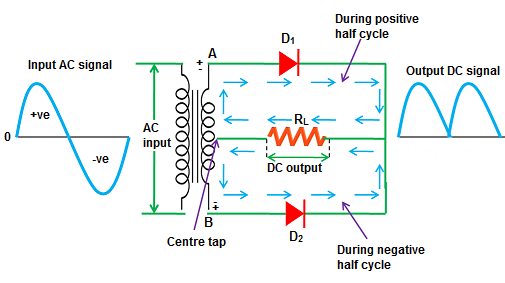# Describe the Full-Wave Bridge Rectifier

The full-wave bridge rectifier is a commonly used rectifier circuit that converts an alternating current (AC) input signal into a pulsating direct current (DC) output signal. It utilizes a combination of diodes arranged in a bridge configuration to achieve full-wave rectification.

Here’s a description of the full-wave bridge rectifier operation:

1. Circuit Configuration:
• The full-wave bridge rectifier consists of four diodes connected in a bridge configuration.
• The AC input signal is applied across the input terminals of the bridge, and the DC output is taken from the output terminals.
2. Operation:
• During the positive half-cycle of the input AC signal, two diodes (D1 and D2) are forward-biased, while the other two diodes (D3 and D4) are reverse-biased.
• The forward-biased diodes provide current paths for the positive half-cycle, allowing current to flow through the load in one direction.
• The current flows through the load resistor and creates a positive voltage across it.
3. Rectification:
• The full-wave bridge rectifier achieves full-wave rectification by utilizing both the positive and negative half-cycles of the input AC signal.
• During the negative half-cycle of the input AC signal, diodes D3 and D4 become forward-biased, while D1 and D2 become reverse-biased.
• The forward-biased diodes provide current paths for the negative half-cycle, allowing current to flow through the load in the opposite direction.
• As a result, the current through the load and the voltage across it are still in the same direction, providing full-wave rectification.
4. Output Waveform:
• The output waveform of the full-wave bridge rectifier is a pulsating DC waveform.
• It consists of a series of positive and negative half-cycles of the input waveform, with both the positive and negative half-cycles rectified.
5. Ripple Voltage:
• The full-wave bridge rectifier has a lower ripple voltage compared to the half-wave rectifier.
• Since it utilizes both halves of the input waveform, the time between successive peaks in the output waveform is reduced, resulting in a smaller ripple voltage.
• However, the ripple voltage still exists due to factors such as the capacitance in the load and the load resistor value.
6. Applications:
• The full-wave bridge rectifier is widely used in various applications where a higher level of rectification and lower ripple voltage are required.
• It is commonly found in power supplies, battery chargers, motor control circuits, and other applications that require efficient and reliable rectification.

The full-wave bridge rectifier provides improved rectification efficiency and a lower ripple voltage compared to the half-wave rectifier. It is a versatile rectifier circuit that is widely used in many electronic devices and power systems.

# Derive the parameters of Full-Wave Centre-Tap and Bridge Rectifiers

The parameters of Full-Wave Centre-Tap and Bridge Rectifiers can be derived as follows:

Full-Wave Centre-Tap Rectifier:

• Average DC Output Voltage (Vdc): Vdc = 2Vm/π, where Vm is the peak value of the sinusoidal AC input voltage.
• Ripple Factor (rf): rf = 1/π * (R / 2R + L / 2πfC), where R is the load resistance, L is the inductance of the centre-tapped transformer, f is the frequency of the AC input voltage, and C is the capacitance of the filter capacitor.
• Efficiency (η): η = Vdc / Vm * 100%

Full-Wave Bridge Rectifier:

• Average DC Output Voltage (Vdc): Vdc = 2Vm/π, where Vm is the peak value of the sinusoidal AC input voltage.
• Ripple Factor (rf): rf = 1/π * (R / 4R + L / πfC), where R is the load resistance, L is the inductance of the input filter, f is the frequency of the AC input voltage, and C is the capacitance of the filter capacitor.
• Efficiency (η): η = Vdc / Vm * 100%

These are the general formulae for the parameters of Full-Wave Centre-Tap and Bridge Rectifiers, but the actual values may vary depending on the specific components and operating conditions of the rectifier circuit.

# Recall Filters

Filters are electronic circuits used to pass certain frequencies or frequency ranges while blocking others. They are used to modify or shape the frequency response of a signal, either by attenuating or amplifying certain frequencies or by removing unwanted frequencies. Filters are commonly used in audio and radio frequency applications, and in power supplies to remove ripple and noise.

Filters can be classified into two categories: passive filters and active filters. Passive filters use passive components, such as resistors, capacitors, and inductors, to attenuate or amplify certain frequencies. Active filters use active components, such as operational amplifiers, to provide gain and filtering.

Passive Filters:

Passive filters are the simplest type of filter and are constructed using only passive components. There are four basic types of passive filters: high-pass, low-pass, band-pass, and band-stop filters.

1. High-pass filter:

A high-pass filter allows high-frequency signals to pass through while blocking low-frequency signals. It is constructed using a capacitor and a resistor. The capacitor blocks low-frequency signals, while the resistor allows high-frequency signals to pass through.

1. Low-pass filter:

A low-pass filter allows low-frequency signals to pass through while blocking high-frequency signals. It is constructed using a resistor and a capacitor. The capacitor blocks high-frequency signals, while the resistor allows low-frequency signals to pass through.

1. Band-pass filter:

A band-pass filter allows a specific range of frequencies to pass through while blocking all other frequencies. It is constructed using a combination of capacitors, resistors, and inductors.

1. Band-stop filter:

A band-stop filter, also known as a notch filter, blocks a specific range of frequencies while allowing all other frequencies to pass through. It is constructed using a combination of capacitors, resistors, and inductors.

Active Filters:

Active filters use active components, such as operational amplifiers, to provide gain and filtering. Active filters have several advantages over passive filters, such as higher gain, wider frequency range, and better selectivity.

1. Low-pass filter:

An active low-pass filter uses an operational amplifier to amplify and filter low-frequency signals. It is constructed using a combination of resistors, capacitors, and an operational amplifier.

1. High-pass filter:

An active high-pass filter uses an operational amplifier to amplify and filter high-frequency signals. It is constructed using a combination of resistors, capacitors, and an operational amplifier.

1. Band-pass filter:

An active band-pass filter uses an operational amplifier to amplify and filter a specific range of frequencies. It is constructed using a combination of resistors, capacitors, inductors, and an operational amplifier.

1. Band-stop filter:

An active band-stop filter uses an operational amplifier to amplify and filter a specific range of frequencies. It is constructed using a combination of resistors, capacitors, inductors, and an operational amplifier.

In summary, filters are electronic circuits used to pass certain frequencies or frequency ranges while blocking others. There are two basic types of filters: passive filters and active filters. Passive filters use passive components, such as resistors, capacitors, and inductors, while active filters use active components, such as operational amplifiers, to provide gain and filtering. There are four basic types of passive filters: high-pass, low-pass, band-pass, and band-stop filters, while active filters can be designed to provide any of these four types of filtering.

# Describe the working of Capacitor Filter

A capacitor filter, also known as a smoothing capacitor or a reservoir capacitor, is a circuit component used to reduce the ripple voltage in a rectified output waveform. It is commonly used in power supply circuits to convert pulsating DC into a more stable and smoother DC voltage.

Here’s a description of the working of a capacitor filter:

1. Circuit Configuration:
• The capacitor filter is typically connected in parallel to the load resistor in a rectifier circuit.
• The rectifier circuit can be either a half-wave or a full-wave rectifier, which converts an AC input signal into a pulsating DC output.
2. Charging Phase:
• During the charging phase of the capacitor, the rectifier circuit charges the capacitor to its peak voltage.
• In the case of a half-wave rectifier, the capacitor charges during the positive half-cycle of the input waveform, while in a full-wave rectifier, it charges during both the positive and negative half-cycles.
• The capacitor charges up quickly when the rectified voltage exceeds its current voltage level.
3. Discharging Phase:
• During the discharging phase, when the rectifier output voltage drops below the capacitor voltage, the capacitor discharges its stored energy to the load resistor.
• The capacitor acts as a temporary energy source, supplying current to the load when the rectified voltage is low or zero.
• This helps to maintain a more constant DC voltage across the load, reducing the ripple voltage.
4. Ripple Voltage Reduction:
• The primary function of the capacitor filter is to reduce the ripple voltage present in the rectified output waveform.
• The capacitor acts as a storage element, smoothing out the variations in the rectified voltage.
• It charges up during the peaks of the rectified voltage and discharges during the low points, effectively reducing the ripple.
5. Selection of Capacitance:
• The capacitance value of the capacitor used in the filter circuit is chosen based on the desired level of ripple reduction.
• A larger capacitance value results in better ripple reduction but may require larger physical size and have slower response times.
• Conversely, a smaller capacitance value provides less ripple reduction but offers faster response times and smaller physical size.
6. Application:
• Capacitor filters are widely used in power supply circuits to provide a smoother DC voltage to electronic devices.
• They are essential in eliminating or reducing the ripple voltage, which can cause interference and affect the performance of sensitive electronic components.

By storing and releasing energy during the charging and discharging phases, a capacitor filter smooths out the pulsating DC voltage produced by rectifiers, resulting in a more stable and constant DC voltage across the load. It helps to reduce ripple voltage and ensures the proper functioning of electronic devices powered by the rectified signal.

# Calculate the Ripple Factor of Capacitor Filter

The Ripple Factor of a Capacitor Filter is a measure of the amount of AC voltage ripple present in the filtered DC output. It is defined as the ratio of the root mean square (RMS) value of the AC component of the output voltage to the average DC output voltage. The formula for the ripple factor of a Capacitor Filter is:

Ripple Factor (rf) = √(Vrms2 / Vdc2)

Where:

Vrms = the RMS value of the AC component of the output voltage

Vdc = the average DC output voltage

To calculate the ripple factor, the RMS value of the AC component of the output voltage must be found first. This can be done by measuring the output voltage waveform and finding its RMS value, or by using simulation software to model the circuit and obtain the RMS value of the output voltage.

Once the RMS value of the AC component is known, the average DC output voltage can be found by measuring the DC output voltage or by using simulation software. The ratio of the RMS value of the AC component to the average DC output voltage is then calculated to find the ripple factor. The lower the ripple factor, the smoother the DC output voltage, and the better the filtering performance of the capacitor filter.

# Describe the working of Inductor Filter

An inductor filter, also known as a choke or a smoothing inductor, is a circuit component used to filter out high-frequency noise and ripple in a power supply circuit. It is commonly used in conjunction with capacitors to create a low-pass filter and produce a smoother DC voltage output.

Here’s a description of the working of an inductor filter:

1. Circuit Configuration:
• The inductor filter is typically connected in series with the load resistor in a power supply circuit.
• It is placed between the rectifier output and the load resistor, along with a capacitor connected in parallel to the load.
2. Inductor Operation:
• When an AC signal is applied to the inductor, it generates a magnetic field around itself.
• The inductor opposes changes in current, storing energy in its magnetic field when the current increases and releasing energy when the current decreases.
3. Filtering Operation:
• The inductor filter acts as a low-pass filter, allowing low-frequency components (DC) of the input signal to pass through while attenuating or blocking high-frequency components (ripple and noise).
• The inductor, together with the capacitor, forms a filter network that smooths out the rectified output waveform by reducing the rate of change of current.
4. Ripple Reduction:
• When the rectified voltage drops during the low points of the input waveform, the inductor releases its stored energy to the load.
• This helps to maintain a more constant DC voltage across the load by reducing the amplitude of the ripple voltage.
5. Inductor Characteristics:
• The inductor’s ability to filter out high-frequency noise depends on its inductance value and the load impedance.
• Higher inductance values provide better filtering performance, while lower values may allow more ripple to pass through.
• The inductor’s series resistance (DC resistance) also affects the filter’s performance and should be kept as low as possible.
6. Application:
• Inductor filters are commonly used in power supply circuits where a smoother DC voltage is required.
• They are effective in reducing high-frequency noise and ripple caused by rectification, switching, or other sources of interference.
• Inductor filters find applications in various electronic devices and systems, including audio equipment, communication systems, and power converters.

By utilizing the inductor’s energy storage and release characteristics, an inductor filter smooths out the rectified waveform by reducing ripple and high-frequency noise. It works in conjunction with a capacitor to create a low-pass filter, allowing the DC component to pass while attenuating unwanted high-frequency components. This helps to provide a more stable and clean DC voltage to the load.

# Calculate the Ripple Factor of Inductor Filter

The Ripple Factor of an Inductor Filter is a measure of the amount of AC voltage ripple present in the filtered DC output. It is defined as the ratio of the root mean square (RMS) value of the AC component of the output voltage to the average DC output voltage. The formula for the ripple factor of an Inductor Filter is:

Ripple Factor (rf) = √(Vrms2 / Vdc2)

Where:

Vrms = the RMS value of the AC component of the output voltage

Vdc = the average DC output voltage

To calculate the ripple factor, the RMS value of the AC component of the output voltage must be found first. This can be done by measuring the output voltage waveform and finding its RMS value, or by using simulation software to model the circuit and obtain the RMS value of the output voltage.

Once the RMS value of the AC component is known, the average DC output voltage can be found by measuring the DC output voltage or by using simulation software. The ratio of the RMS value of the AC component to the average DC output voltage is then calculated to find the ripple factor. The lower the ripple factor, the smoother the DC output voltage, and the better the filtering performance of the inductor filter.

# Describe the working of LC Filter

An LC filter, also known as an L-C filter or a tank circuit, is a type of electronic filter that utilizes the combination of an inductor (L) and a capacitor (C) to filter out unwanted frequencies from a signal. It is commonly used in various applications, including power supplies and radio frequency (RF) circuits.

Here’s a description of the working of an LC filter:

1. Circuit Configuration:
• The LC filter consists of an inductor (L) and a capacitor (C) connected in parallel or in series, depending on the desired filter response.
• The input signal is applied to the LC filter, and the filtered output is taken across the inductor or capacitor.
2. Resonant Frequency:
• The LC filter operates based on the principle of resonance, where the inductor and capacitor resonate at a specific frequency called the resonant frequency (fr).
• The resonant frequency is determined by the values of the inductor and capacitor and is given by the equation fr = 1 / (2π√(LC)).
3. Filter Operation:
• At the resonant frequency, the reactance of the inductor and capacitor cancel each other out, resulting in a low impedance path for the desired frequency.
• This allows the desired frequency component of the input signal to pass through the filter with minimum loss.
4. Frequency Selectivity:
• The LC filter provides frequency selectivity, allowing signals near the resonant frequency to pass through while attenuating signals at other frequencies.
• The bandwidth of the filter, known as the passband, is determined by the quality factor (Q) of the LC circuit, which is related to the resistance and energy loss in the circuit.
5. High-Frequency Attenuation:
• As the frequency moves away from the resonant frequency, the impedance of the LC circuit increases, causing a higher attenuation of the signal.
• This results in effective filtering of high-frequency components, reducing their amplitudes in the output signal.
6. Applications:
• LC filters are widely used in power supply circuits to reduce noise and ripple in the output voltage.
• They are also employed in RF circuits to select specific frequency bands or reject unwanted frequencies.
• LC filters can be found in audio systems, radio receivers, transmitters, and other electronic devices requiring frequency filtering.

By utilizing the resonant behavior of the inductor and capacitor, an LC filter allows signals near the resonant frequency to pass through with minimal loss while attenuating signals at other frequencies. It provides frequency selectivity and can effectively filter out unwanted frequencies, making it a valuable component in various electronic circuits.

# Describe the working of CLC or π-Filter

A CLC or π-filter is a type of LC filter configuration that combines capacitors (C) and inductors (L) to achieve enhanced filtering characteristics. It is commonly used in power supply circuits to provide a cleaner and more stable DC voltage output.

Here’s a description of the working of a CLC or π-filter:

1. Circuit Configuration:
• A CLC or π-filter consists of an input capacitor (C1), an inductor (L), and an output capacitor (C2) connected in a specific arrangement.
• The inductor is placed between the two capacitors, forming a π-shaped configuration.
2. Filtering Operation:
• The input capacitor (C1) blocks high-frequency noise and ripple present in the input signal, preventing them from reaching the inductor.
• The inductor (L) provides impedance to the high-frequency components, effectively filtering them out.
• The output capacitor (C2) further smoothes the filtered signal by storing and releasing charge to maintain a stable DC voltage.
3. Low-Pass Filtering:
• The CLC or π-filter primarily acts as a low-pass filter, allowing low-frequency components (DC) to pass through while attenuating high-frequency noise and ripple.
• The combination of the inductor and capacitors creates a resonant circuit that selectively filters out high-frequency components.
4. Improved Filtering:
• The CLC or π-filter configuration offers improved filtering characteristics compared to a simple LC filter.
• The input capacitor (C1) provides additional noise rejection and prevents the inductor from directly sensing the input ripple.
• The output capacitor (C2) provides additional smoothing, reducing the ripple voltage further.
5. Ripple Reduction:
• The CLC or π-filter effectively reduces the ripple voltage present in the power supply output.
• The inductor and capacitors work together to store and release energy, ensuring a more stable and constant DC voltage across the load.
6. Applications:
• CLC or π-filters are commonly used in power supply circuits to provide clean DC voltage to electronic devices.
• They are particularly effective in reducing ripple and noise caused by rectification and other sources of interference.
• CLC or π-filters can be found in various electronic equipment, including audio amplifiers, computer power supplies, and industrial power systems.

By combining capacitors and an inductor in a specific arrangement, the CLC or π-filter configuration enhances the filtering characteristics of an LC filter. It effectively filters out high-frequency noise and ripple, providing a smoother and more stable DC voltage output. The CLC or π-filter is a popular choice in power supply applications where clean and reliable DC voltage is required.

# Describe the working of Series Clipper Circuit and draw the output waveform of the Circuit

A series clipper circuit is a type of electronic circuit that clips or cuts off a portion of the input waveform above or below a certain voltage level. It consists of a diode connected in series with the load resistor.

The working of a series clipper circuit can be understood as follows:

1. Circuit Configuration:
• The series clipper circuit consists of an input signal source, a diode, and a load resistor connected in series.
• The diode is oriented in such a way that its cathode is connected towards the load resistor and its anode is connected towards the input signal source.
2. Positive Series Clipper:
• In a positive series clipper, the diode is forward biased when the input voltage is above a certain threshold voltage (Vth).
• When the input voltage is higher than Vth, the diode conducts and behaves like a closed switch, allowing current to flow through the load resistor.
• As a result, the output voltage across the load resistor is approximately equal to the forward-biased voltage drop across the diode.
3. Negative Series Clipper:
• In a negative series clipper, the diode is reverse biased when the input voltage is below a certain threshold voltage (Vth).
• When the input voltage is lower than Vth, the diode acts as an open switch and does not conduct.
• In this case, the output voltage across the load resistor is approximately equal to the input voltage.
4. Output Waveform:
• The output waveform of a series clipper circuit depends on the orientation of the diode and the input signal.
• In a positive series clipper, the portion of the input waveform above Vth is clipped off, resulting in a truncated waveform with the top portion clipped at Vth.
• In a negative series clipper, the portion of the input waveform below Vth is clipped off, resulting in a truncated waveform with the bottom portion clipped at Vth.
• The clipped portion of the waveform appears flat or clipped due to the diode’s action of blocking or conducting current.

The output waveform of a series clipper circuit can be illustrated as follows:

For a positive series clipper: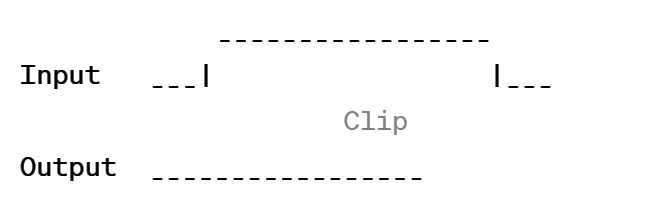For a negative series clipper: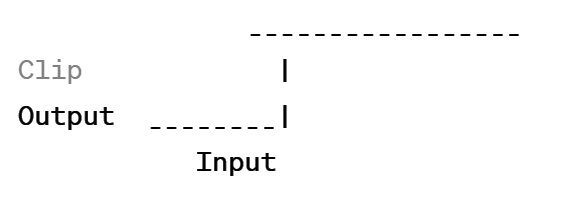The output waveform will have a portion clipped or cut off, depending on the orientation of the diode and the voltage level at which it conducts or blocks current. The clipped portion of the waveform will appear flat or clipped at the threshold voltage.

# Describe the working of Shunt Clipper Circuit and draw the output waveform of the Circuit

A shunt clipper circuit, also known as a parallel clipper circuit, is a type of electronic circuit that clips or cuts off a portion of the input waveform by diverting current through a shunt branch when the voltage exceeds a certain threshold. It consists of a diode connected in parallel with the load resistor.

The working of a shunt clipper circuit can be understood as follows:

1. Circuit Configuration:
• The shunt clipper circuit consists of an input signal source, a diode, and a load resistor connected in parallel.
• The diode is oriented in such a way that its cathode is connected towards the load resistor and its anode is connected towards the ground or reference potential.
2. Positive Shunt Clipper:
• In a positive shunt clipper, the diode is reverse biased when the input voltage is below a certain threshold voltage (Vth).
• When the input voltage is lower than Vth, the diode acts as an open switch and does not conduct.
• In this case, the output voltage across the load resistor is approximately equal to the input voltage.
3. Negative Shunt Clipper:
• In a negative shunt clipper, the diode is forward biased when the input voltage is above a certain threshold voltage (Vth).
• When the input voltage is higher than Vth, the diode conducts and behaves like a closed switch, diverting current through the diode branch.
• As a result, the output voltage across the load resistor is clamped to the threshold voltage (Vth) as the excess current is shunted away through the diode branch.
4. Output Waveform:
• The output waveform of a shunt clipper circuit depends on the orientation of the diode and the input signal.
• In a positive shunt clipper, the portion of the input waveform below Vth is clipped off, resulting in a truncated waveform with the bottom portion clipped at Vth.
• In a negative shunt clipper, the portion of the input waveform above Vth is clipped off, resulting in a truncated waveform with the top portion clipped at Vth.
• The clipped portion of the waveform appears flat or clipped due to the diode’s action of diverting current away from the load resistor.

The output waveform of a shunt clipper circuit can be illustrated as follows:

For a positive shunt clipper: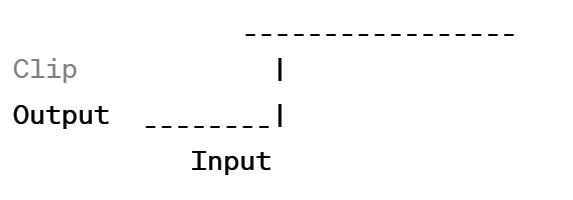For a negative shunt clipper: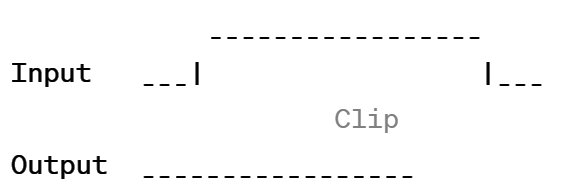The output waveform will have a portion clipped or cut off, depending on the orientation of the diode and the voltage level at which it conducts or blocks current. The clipped portion of the waveform will appear flat or clipped at the threshold voltage.

# Describe the working of Shunt-Biased Clipper Circuit and draw the output waveform of the Circuit

A shunt-biased clipper circuit is a type of electronic circuit that combines the functionalities of both a shunt clipper and a biasing resistor to clip or cut off a portion of the input waveform. It consists of a diode connected in parallel with the load resistor, along with a biasing resistor.

The working of a shunt-biased clipper circuit can be understood as follows:

1. Circuit Configuration:
• The shunt-biased clipper circuit consists of an input signal source, a diode, a load resistor, and a biasing resistor connected in parallel.
• The diode is oriented in such a way that its cathode is connected towards the load resistor and its anode is connected towards the ground or reference potential.
• The biasing resistor is connected in series with the diode and the load resistor.
2. Biasing Effect:
• The biasing resistor introduces a voltage drop across itself due to the flow of current through it.
• This voltage drop biases the diode and changes its forward or reverse bias conditions based on the polarity of the input signal.
3. Positive Shunt-Biased Clipper:
• In a positive shunt-biased clipper, when the input voltage is below the biasing voltage (Vbias), the diode is reverse biased.
• When the diode is reverse biased, it acts as an open switch and does not conduct.
• In this case, the output voltage across the load resistor is approximately equal to the input voltage.
4. Negative Shunt-Biased Clipper:
• In a negative shunt-biased clipper, when the input voltage is above the biasing voltage (Vbias), the diode is forward biased.
• When the diode is forward biased, it conducts and behaves like a closed switch, diverting current through the diode branch.
• As a result, the output voltage across the load resistor is clamped to the biasing voltage (Vbias) as the excess current is shunted away through the diode branch.
5. Output Waveform:
• The output waveform of a shunt-biased clipper circuit depends on the orientation of the diode, the biasing voltage, and the input signal.
• In a positive shunt-biased clipper, the portion of the input waveform below the biasing voltage (Vbias) is clipped off, resulting in a truncated waveform with the bottom portion clipped at Vbias.
• In a negative shunt-biased clipper, the portion of the input waveform above the biasing voltage (Vbias) is clipped off, resulting in a truncated waveform with the top portion clipped at Vbias.
• The clipped portion of the waveform appears flat or clipped due to the diode’s action of diverting current away from the load resistor.

The output waveform of a shunt-biased clipper circuit can be illustrated as follows:

For a positive shunt-biased clipper: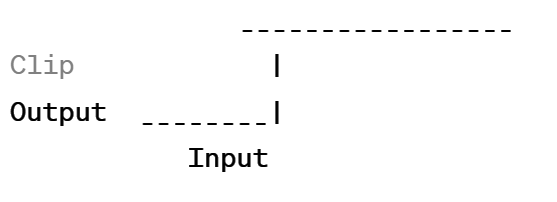For a negative shunt-biased clipper: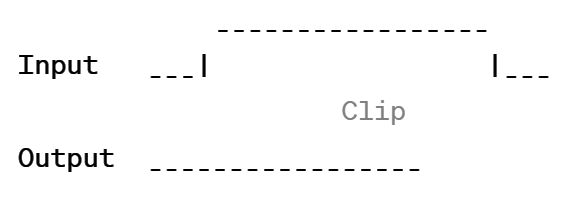The output waveform will have a portion clipped or cut off, depending on the orientation of the diode, the biasing voltage, and the voltage level at which the diode conducts or blocks current. The clipped portion of the waveform will appear flat or clipped at the biasing voltage.

# Describe the working of Series-Biased Clipper Circuit and draw the output waveform of the Circuit

A series-biased clipper circuit is a type of electronic circuit that combines the functionalities of both a series clipper and a biasing resistor to clip or cut off a portion of the input waveform. It consists of a diode connected in series with the load resistor, along with a biasing resistor.

The working of a series-biased clipper circuit can be understood as follows:

1. Circuit Configuration:
• The series-biased clipper circuit consists of an input signal source, a diode, a load resistor, and a biasing resistor connected in series.
• The diode is oriented in such a way that its cathode is connected towards the load resistor and its anode is connected towards the input signal source.
• The biasing resistor is connected in parallel with the diode and the load resistor.
2. Biasing Effect:
• The biasing resistor introduces a voltage drop across itself due to the flow of current through it.
• This voltage drop biases the diode and changes its forward or reverse bias conditions based on the polarity of the input signal.
3. Positive Series-Biased Clipper:
• In a positive series-biased clipper, when the input voltage is above the biasing voltage (Vbias), the diode is forward biased.
• When the diode is forward biased, it conducts and behaves like a closed switch, allowing current to flow through the load resistor.
• As a result, the output voltage across the load resistor is approximately equal to the forward-biased voltage drop across the diode.
4. Negative Series-Biased Clipper:
• In a negative series-biased clipper, when the input voltage is below the biasing voltage (Vbias), the diode is reverse biased.
• When the diode is reverse biased, it acts as an open switch and does not conduct.
• In this case, the output voltage across the load resistor is approximately equal to the input voltage.
5. Output Waveform:
• The output waveform of a series-biased clipper circuit depends on the orientation of the diode, the biasing voltage, and the input signal.
• In a positive series-biased clipper, the portion of the input waveform above the biasing voltage (Vbias) is clipped off, resulting in a truncated waveform with the top portion clipped at Vbias.
• In a negative series-biased clipper, the portion of the input waveform below the biasing voltage (Vbias) is clipped off, resulting in a truncated waveform with the bottom portion clipped at Vbias.
• The clipped portion of the waveform appears flat or clipped due to the diode’s action of blocking or conducting current.

The output waveform of a series-biased clipper circuit can be illustrated as follows:

For a positive series-biased clipper: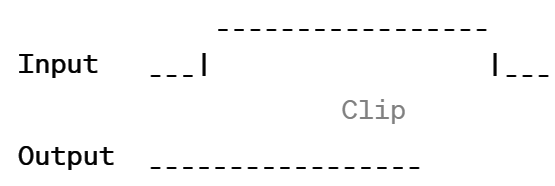For a negative series-biased clipper: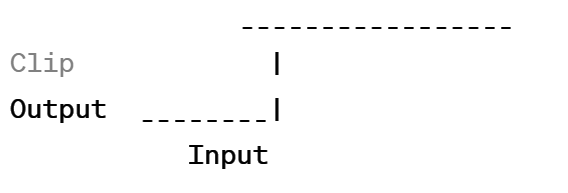The output waveform will have a portion clipped or cut off, depending on the orientation of the diode, the biasing voltage, and the voltage level at which the diode conducts or blocks current. The clipped portion of the waveform will appear flat or clipped at the biasing voltage.

# Recall the Combinational Clipper Circuit

A Combinational Clipper Circuit is a type of waveform shaping circuit that combines the features of both Series and Shunt Clipper Circuits. The circuit is designed to clip a portion of the input waveform, with the clipping level and waveform shape determined by the combination of the series and shunt clipping elements.

In a Combinational Clipper Circuit, the input waveform is applied to both a Series Clipper Circuit and a Shunt Clipper Circuit, with the outputs of both circuits combined to create the final output waveform. This allows for greater control over the clipping level and waveform shape compared to a single Series or Shunt Clipper Circuit.

The Combinational Clipper Circuit is used in a variety of applications, including waveform shaping, clipping of over-voltages, and limiting the magnitude of signals. The circuit can be designed to produce a variety of waveform shapes, including symmetrical and asymmetrical waveforms, and can be used to create waveforms with steep edges or with gradual transitions between the clipped and unclipped portions.

# Draw the Output Waveform of the Clipper Circuit

The output waveform of a Combinational Clipper Circuit will depend on the specific design of the circuit and the input waveform applied to it. However, generally speaking, the output waveform will have the shape of the input waveform, but with a portion of it clipped or removed.

For example, if the input waveform is a sinusoidal wave with positive and negative peaks, the output waveform may have the positive peaks clipped at a certain level, while the negative peaks are left unchanged. This results in an asymmetrical waveform with steep edges at the transition between the clipped and unclipped portions.

Positive clipper circuit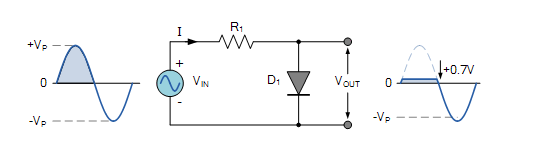Negative Clipper circuit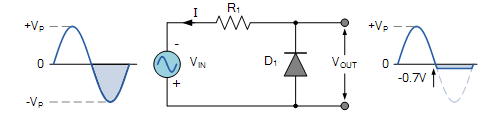# Recall the Clamper Circuits and their Types

Clamper circuits are electronic circuits that are used to add or subtract a DC voltage level to an AC signal. These circuits consist of a capacitor, a diode, and a resistor. The capacitor is used to store charge and the diode is used to control the direction of the current flow. The resistor is used to limit the current flow through the circuit.

There are two types of clamper circuits:

1. Positive Clamper Circuit
2. Negative Clamper Circuit
3. Positive Clamper Circuit:

The positive clamper circuit is used to add a positive DC voltage to an AC signal. In this circuit, the capacitor is connected in series with the load and the diode is connected in parallel with the load. When the input signal is negative, the diode is reverse-biased and the capacitor is charged to the peak value of the input signal. When the input signal becomes positive, the diode is forward-biased and the capacitor discharges through the load, adding the voltage of the capacitor to the input signal.

1. Negative Clamper Circuit:

The negative clamper circuit is used to subtract a negative DC voltage from an AC signal. In this circuit, the capacitor is connected in parallel with the load and the diode is connected in series with the load. When the input signal is positive, the diode is forward-biased and the capacitor charges to the peak value of the input signal. When the input signal becomes negative, the diode is reverse-biased and the capacitor discharges through the load, subtracting the voltage of the capacitor from the input signal.

Clamper circuits find applications in various electronic devices such as television sets, radios, and other electronic equipment. They are also used in signal processing, pulse shaping, and communication systems.

# Describe the working of Positive Clamper Circuit and draw the output waveform of the Circuit

A positive clamper circuit, also known as a positive DC restorer circuit, is an electronic circuit that adds a DC voltage to an input waveform to shift it upwards. It allows the positive peaks of the input waveform to be clamped at a desired voltage level.

The working of a positive clamper circuit can be understood as follows:

1. Circuit Configuration:
• The positive clamper circuit consists of an input signal source, a capacitor, a diode, and a load resistor.
• The capacitor is connected in series with the input signal source and the diode, and the load resistor is connected in parallel with the diode.
• The diode is oriented in such a way that its cathode is connected towards the capacitor and its anode is connected towards the load resistor.
2. Charging Phase:
• Initially, when the input voltage is below the clamping voltage, the diode is reverse biased, and no current flows through the diode.
• During this phase, the capacitor charges up to the peak value of the input voltage through the input signal source.
3. Clamping Phase:
• When the input voltage rises above the clamping voltage, the diode becomes forward biased, allowing current to flow through the diode.
• As a result, the capacitor becomes fully charged to the peak value of the input voltage plus the forward voltage drop across the diode.
• The diode acts as a closed switch, clamping the voltage across the load resistor to the voltage across the capacitor.
• The clamping voltage is determined by the voltage level of the input signal source and the forward voltage drop across the diode.
4. Output Waveform:
• The output waveform of a positive clamper circuit is obtained by shifting the input waveform upwards by the clamping voltage.
• The positive peaks of the input waveform are clamped to the clamping voltage, while the negative peaks are unaffected.
• The output waveform retains the same shape as the input waveform, but it is shifted vertically.
• The amplitude of the output waveform is higher than the input waveform by the clamping voltage.

The output waveform of a positive clamper circuit can be illustrated as follows: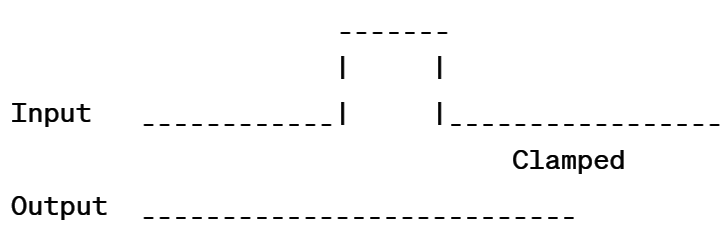The output waveform will have the positive peaks clamped at the clamping voltage, while the negative peaks remain unchanged. The clamped portion of the waveform appears flat at the clamping voltage level.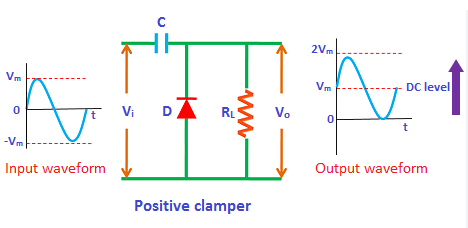# Describe the working of Negative Clamper Circuit and draw the output waveform of the Circuit

A negative clamper circuit, also known as a negative DC restorer circuit, is an electronic circuit that adds a DC voltage to an input waveform to shift it downwards. It allows the negative peaks of the input waveform to be clamped at a desired voltage level.

The working of a negative clamper circuit can be understood as follows:

1. Circuit Configuration:
• The negative clamper circuit consists of an input signal source, a capacitor, a diode, and a load resistor.
• The capacitor is connected in series with the input signal source and the diode, and the load resistor is connected in parallel with the diode.
• The diode is oriented in such a way that its anode is connected towards the capacitor and its cathode is connected towards the load resistor.
2. Charging Phase:
• Initially, when the input voltage is above the clamping voltage, the diode is reverse biased, and no current flows through the diode.
• During this phase, the capacitor charges up to the peak value of the input voltage through the input signal source.
3. Clamping Phase:
• When the input voltage drops below the clamping voltage, the diode becomes forward biased, allowing current to flow through the diode.
• As a result, the capacitor becomes fully charged to the peak value of the input voltage plus the forward voltage drop across the diode.
• The diode acts as a closed switch, clamping the voltage across the load resistor to the voltage across the capacitor.
• The clamping voltage is determined by the voltage level of the input signal source and the forward voltage drop across the diode.
4. Output Waveform:
• The output waveform of a negative clamper circuit is obtained by shifting the input waveform downwards by the clamping voltage.
• The negative peaks of the input waveform are clamped to the clamping voltage, while the positive peaks are unaffected.
• The output waveform retains the same shape as the input waveform, but it is shifted vertically.
• The amplitude of the output waveform is lower than the input waveform by the clamping voltage.

The output waveform of a negative clamper circuit can be illustrated as follows: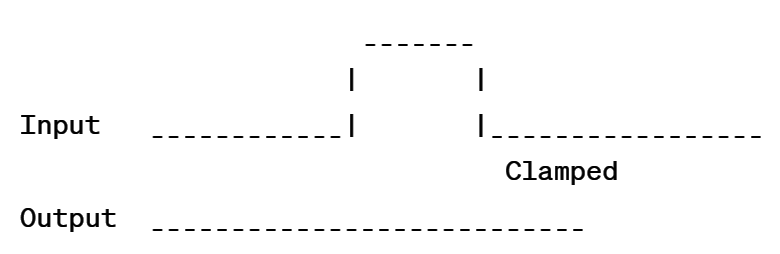The output waveform will have the negative peaks clamped at the clamping voltage, while the positive peaks remain unchanged. The clamped portion of the waveform appears flat at the clamping voltage level.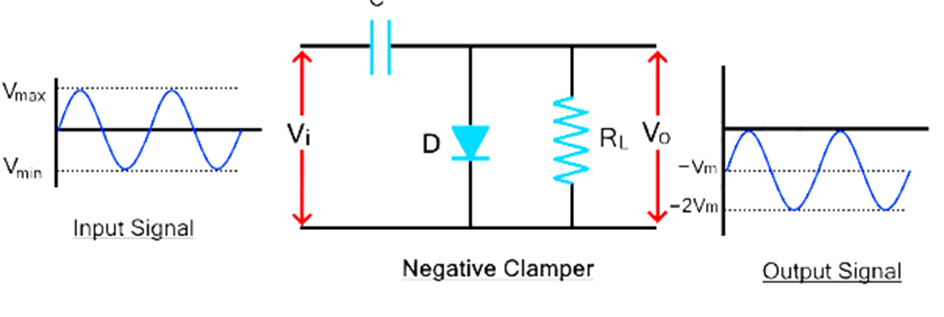# Recall the biased Clamper Circuits

A biased Clamper Circuit is a combination of a clipper and a clamper circuit, designed to add or remove a DC offset from an AC waveform. biased Clamper Circuits can be positive or negative, depending on the direction of the DC offset.

A positive biased clamper circuit adds a positive DC offset to an AC waveform, resulting in a waveform that swings positive and negative about a positive DC level. A negative biased clamper circuit adds a negative DC offset to an AC waveform, resulting in a waveform that swings positive and negative about a negative DC level.

The working of a biased Clamper Circuit can be explained as follows:

1. The AC waveform is applied to the input of the circuit.
2. The clipper part of the circuit is used to limit the amplitude of the waveform, while the clamper part is used to add or remove the DC offset.
3. The output waveform has the same shape as the input waveform, but with the addition of a DC offset.

The output waveform of a biased Clamper Circuit will depend on the specific design of the circuit, as well as the input waveform and the desired DC offset. For example, if the input waveform is a sinusoidal wave, the output waveform will also be a sinusoidal wave, but with the addition of a positive or negative DC offset.

# Draw the output waveform of Biased Clamper Circuits

A biased clamper circuit, also known as a DC-restored clamped circuit, is an electronic circuit that removes the DC component of a waveform while preserving the AC component. The output waveform of a biased clamped circuit depends on the input waveform and the values of the components used in the circuit.

The basic circuit of a biased clamped circuit consists of a diode, a capacitor, a resistor, and a DC bias voltage source. The diode is used to clamp the input waveform at a certain level, while the capacitor and resistor are used to filter out the DC component of the waveform and pass the AC component. The DC bias voltage source is used to set the DC level of the output waveform.

The waveform of the output of a biased clamper circuit can be divided into three parts:

1. Initial transient response: When the input waveform is applied to the circuit, the diode is initially reverse-biased and the capacitor is uncharged. The output waveform during this stage is undefined.
2. Clamping action: As the input waveform increases and becomes positive, the diode becomes forward-biased, and the capacitor charges to the peak value of the input waveform. The output waveform during this stage is a square waveform with the same peak value as the input waveform.
3. Steady-state response: After the initial transient response, the diode remains forward-biased, and the capacitor acts as a low-pass filter, passing the AC component of the input waveform while removing the DC component. The output waveform during this stage is a sinusoidal waveform with the same frequency as the input waveform but with a DC level set by the DC bias voltage source.

In summary, the output waveform of a biased clamped circuit is a square waveform during the clamping action and a sinusoidal waveform during the steady-state response. The exact shape of the output waveform depends on the input waveform, the values of the components used in the circuit, and the DC bias voltage source.

# Recall the Voltage Doubler Circuits

A Voltage Doubler Circuit is an electronic circuit that is used to double the peak voltage of a DC waveform. It is often used to generate a high voltage DC output from a low voltage DC input.

Voltage Doubler Circuits work by storing energy in capacitors during the positive half cycle of the input waveform and then releasing it during the negative half cycle. This results in a DC waveform with twice the peak voltage of the input waveform.

There are two main types of Voltage Doubler Circuits: the single-phase voltage doubler and the two-phase voltage doubler. The single-phase voltage doubler uses one capacitor and one diode, while the two-phase voltage doubler uses two capacitors and two diodes. Both types of circuits provide the same output waveform, but the two-phase voltage doubler is generally more efficient and has a lower output impedance.

# Describe the working of Voltage Doubler Circuits

Voltage doubler circuits are electronic circuits that are used to double the input voltage level. These circuits are commonly used in power supply applications where a higher voltage level is required. There are different types of voltage doubler circuits, such as the Greinacher voltage doubler and the Villard voltage doubler. Here, we will describe the working principle of the Greinacher voltage doubler, which is a popular configuration.

The Greinacher voltage doubler circuit is based on the principle of capacitor charging and discharging. It uses a combination of diodes and capacitors to double the input voltage.

The working of the Greinacher voltage doubler can be understood as follows:

1. Circuit Configuration:
• The Greinacher voltage doubler consists of two stages, each consisting of a diode, a capacitor, and a load resistor.
• The first stage is connected to the input voltage source, and the output of the first stage is connected to the second stage.
• The diodes in each stage are connected in series with opposite polarities, and the capacitors are connected in parallel.
• The load resistors are connected across the capacitors to provide the output voltage.
2. Charging Phase:
• During the positive half-cycle of the input voltage, the diode in the first stage is forward biased, allowing the first capacitor to charge up to the peak value of the input voltage.
• At the same time, the diode in the second stage is reverse biased, preventing the discharge of the second capacitor.
3. Discharging and Doubling Phase:
• During the negative half-cycle of the input voltage, the diode in the first stage is reverse biased, preventing the discharge of the first capacitor.
• However, the diode in the second stage is forward biased, allowing the second capacitor to discharge through the load resistor.
• As a result, the voltage across the second capacitor adds up to the voltage across the first capacitor, effectively doubling the voltage.
4. Output Voltage:
• The output voltage of the voltage doubler circuit is obtained across the load resistor connected to the second capacitor.
• The output voltage is approximately double the peak value of the input voltage, minus the voltage drops across the diodes and the capacitors.
• The output voltage is relatively stable and can be used to power devices that require a higher voltage level.

It is important to note that voltage doubler circuits are suitable for low-current applications due to the limitations of the diodes and capacitors used. Additionally, the output voltage may have some ripple due to the charging and discharging cycles.

Overall, voltage doubler circuits provide a simple and efficient way to double the input voltage level for various applications where higher voltages are required.

# Describe the Voltage Tripler Circuit

A voltage tripler circuit is an electronic circuit that generates an output voltage that is approximately three times the peak value of the input voltage. It is an extension of the voltage doubler circuit, using additional components to achieve a higher voltage multiplication factor. The voltage tripler circuit is commonly used in various applications where a higher voltage level is required.

The working principle of the voltage tripler circuit can be understood as follows:

1. Circuit Configuration:
• The voltage tripler circuit is based on the Greinacher voltage doubler circuit.
• It consists of three stages, each comprising a diode, a capacitor, and a load resistor.
• The diodes in each stage are connected in series with opposite polarities, and the capacitors are connected in parallel.
• The load resistors are connected across the capacitors to provide the output voltage.
2. Charging Phase:
• During the positive half-cycle of the input voltage, the diode in the first stage is forward biased, allowing the first capacitor to charge up to the peak value of the input voltage.
• Simultaneously, the diodes in the second and third stages are reverse biased, preventing the discharge of the second and third capacitors.
3. Discharging and Tripling Phase:
• During the negative half-cycle of the input voltage, the diode in the first stage is reverse biased, preventing the discharge of the first capacitor.
• The diode in the second stage is forward biased, allowing the second capacitor to discharge through the load resistor.
• This discharging process causes the voltage across the second capacitor to add up to twice the voltage across the first capacitor.
• Similarly, during the next negative half-cycle, the diode in the second stage is reverse biased, preventing the discharge of the second capacitor.
• The diode in the third stage is forward biased, allowing the third capacitor to discharge through the load resistor.
• This discharging process causes the voltage across the third capacitor to add up to twice the voltage across the second capacitor.
• As a result, the output voltage across the load resistor connected to the third capacitor is approximately three times the peak value of the input voltage.

The output voltage of the voltage tripler circuit is higher than that of the voltage doubler circuit. However, similar to the voltage doubler circuit, the output voltage may have some ripple due to the charging and discharging cycles. Additionally, the voltage tripler circuit is suitable for low-current applications due to the limitations of the diodes and capacitors used.

In summary, the voltage tripler circuit provides an efficient method to triple the input voltage level, making it useful in various applications that require higher voltage levels.

# Describe the working of Voltage Quadrupler Circuit

A voltage quadrupler circuit is an electronic circuit that generates an output voltage that is approximately four times the peak value of the input voltage. It is an extension of the voltage doubler circuit, using additional components to achieve a higher voltage multiplication factor. The voltage quadrupler circuit is commonly used in applications where a higher voltage level is required.

The working principle of the voltage quadrupler circuit can be understood as follows:

1. Circuit Configuration:
• The voltage quadrupler circuit is based on the Greinacher voltage doubler circuit.
• It consists of four stages, each comprising a diode, a capacitor, and a load resistor.
• The diodes in each stage are connected in series with opposite polarities, and the capacitors are connected in parallel.
• The load resistors are connected across the capacitors to provide the output voltage.
2. Charging Phase:
• During the positive half-cycle of the input voltage, the diode in the first stage is forward biased, allowing the first capacitor to charge up to the peak value of the input voltage.
• Simultaneously, the diodes in the second, third, and fourth stages are reverse biased, preventing the discharge of the second, third, and fourth capacitors.
• During the negative half-cycle of the input voltage, the diode in the first stage is reverse biased, preventing the discharge of the first capacitor.
• The diode in the second stage is forward biased, allowing the second capacitor to discharge through the load resistor.
• This discharging process causes the voltage across the second capacitor to add up to twice the voltage across the first capacitor.
• Similarly, during the next negative half-cycle, the diode in the second stage is reverse biased, preventing the discharge of the second capacitor.
• The diode in the third stage is forward biased, allowing the third capacitor to discharge through the load resistor.
• This discharging process causes the voltage across the third capacitor to add up to twice the voltage across the second capacitor.
• Finally, during the third negative half-cycle, the diode in the third stage is reverse biased, preventing the discharge of the third capacitor.
• The diode in the fourth stage is forward biased, allowing the fourth capacitor to discharge through the load resistor.
• This discharging process causes the voltage across the fourth capacitor to add up to twice the voltage across the third capacitor.
• As a result, the output voltage across the load resistor connected to the fourth capacitor is approximately four times the peak value of the input voltage.

The output voltage of the voltage quadrupler circuit is higher than that of the voltage doubler circuit. However, similar to the voltage doubler circuit, the output voltage may have some ripple due to the charging and discharging cycles. Additionally, the voltage quadrupler circuit is suitable for low-current applications due to the limitations of the diodes and capacitors used.

In summary, the voltage quadrupler circuit provides an efficient method to quadruple the input voltage level, making it useful in various applications that require higher voltage levels.